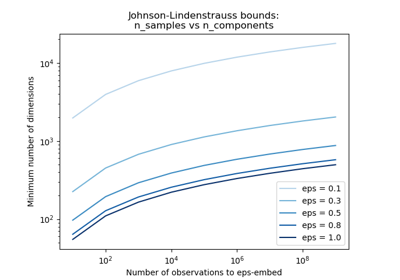sklearn.random_projection.johnson_lindenstrauss_min_dim¶

sklearn.random_projection.johnson_lindenstrauss_min_dim(n_samples, eps=0.1)[source]

Find a ‘safe’ number of components to randomly project to

The distortion introduced by a random projection p only changes the distance between two points by a factor (1 +- eps) in an euclidean space with good probability. The projection p is an eps-embedding as defined by:

(1 - eps) ||u - v||^2 < ||p(u) - p(v)||^2 < (1 + eps) ||u - v||^2

Where u and v are any rows taken from a dataset of shape [n_samples, n_features], eps is in ]0, 1[ and p is a projection by a random Gaussian N(0, 1) matrix with shape [n_components, n_features] (or a sparse Achlioptas matrix).

The minimum number of components to guarantee the eps-embedding is given by:

n_components >= 4 log(n_samples) / (eps^2 / 2 - eps^3 / 3)

Note that the number of dimensions is independent of the original number of features but instead depends on the size of the dataset: the larger the dataset, the higher is the minimal dimensionality of an eps-embedding.

Read more in the User Guide.

Parameters: n_samples : int or numpy array of int greater than 0, Number of samples. If an array is given, it will compute a safe number of components array-wise. eps : float or numpy array of float in ]0,1[, optional (default=0.1) Maximum distortion rate as defined by the Johnson-Lindenstrauss lemma. If an array is given, it will compute a safe number of components array-wise. n_components : int or numpy array of int, The minimal number of components to guarantee with good probability an eps-embedding with n_samples.

References

  Sanjoy Dasgupta and Anupam Gupta, 1999, “An elementary proof of the Johnson-Lindenstrauss Lemma.” http://citeseer.ist.psu.edu/viewdoc/summary?doi=10.1.1.45.3654

Examples

>>> johnson_lindenstrauss_min_dim(1e6, eps=0.5)
663
>>> johnson_lindenstrauss_min_dim(1e6, eps=[0.5, 0.1, 0.01])
array([    663,   11841, 1112658])
>>> johnson_lindenstrauss_min_dim([1e4, 1e5, 1e6], eps=0.1)
array([ 7894,  9868, 11841])

Examples using sklearn.random_projection.johnson_lindenstrauss_min_dim¶The Johnson-Lindenstrauss bound for embedding with random projections